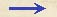Discorsi Propositions 1/06-th-06 Discorsi Proposition1/06-th-06{196} THEOREMA VI, PROPOSITIO VI. {196} THEOREM VI, PROPOSITION VISi duo mobilia aequabili motu ferantur, ratio velocitatum ipsorum composita erit ex ratione spatiorum peractorum et ex ratione temporum contrarie sumptorum. If two particles are carried at a uniform rate, the ratio of their speeds will be the product of the ratio of the distances traversed by the inverse ratio of the time-intervals occupied. Sint duo mobilia A, B, aequabili motu lata; sint autem spatia ab illis peracta in ratione V ad T, tempora vero sint ut S ad R: dico, velocitatem mobilis A ad velocitatem ipsius B habere rationem compositam ex ratione spatii V {10} ad spatium T et temporis R ad tempus S.Sit velocitas C ea cum qua mobile A conficit spatium V in tempore S, et quam rationem habet spatium V ad spatium T, hanc habeat velocitas C ad aliam E; erit E velocitas cum qua mobile B conficit spatium T in tempore eodem S: quod si fiat, ut tempus R ad tempus S, ita velocitas E ad aliam G, erit velocitas G illa secundum quam mobile B conficit spatium T in tempore R. Habemus itaque velocitatem C, cum qua mobile A conficit spatium V in tempore S, et velocitatem G, cum qua mobile B conficit spatium T in tempore R, et est ratio C ad G composita ex rationibus C ad E et E ad G; {20} ratio autem C ad E posita est eadem cum ratione spatii V ad spatium T; ratio vero E ad G est eadem cum ratione R ad S: ergo patet propositum. Let A and B be the two particles which move at a uniform rate; and let the the respective distances traversed by them have the ratio of V to T, but let the time-intervals be as S to R. Then I say the speed of A will bear to the speed of B a ratio which is the product of the ratio of the distance V to the distance T and the time-interval R to the time-interval S. Let C be the speed at which A traverses the distance V during the time-interval S; and let the speed C bear the same ratio to another speed E as V bears to T; then E will be the speed at which B traverses the distance T during the time-interval S. If now the speed E is to another speed G as the time-interval R is to the time-interval S, then G will be the speed at which the particle B traverses the distance T during the time-interval R. Thus we have the speed C at which the particle A covers the distance V during the time S and also the speed G at which the particle B traverses the distance T during the time R. The ratio of C to G is the product of the ratio C to E and E to G; the ratio of C to E is by definition the same as the ratio of the distance V to distance T; and the ratio of E to G is the same as the ratio of R to S. Hence follows the proposition.Discorsi Propositions 1/06-th-06 Discorsi Proposition1/06-th-06